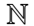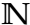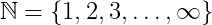# How to write a natural numbers(ℕ) symbol in LaTeX?

Natural numbers are a set of positive numbers from 1 to ∞. Which is represented by symbol. And there is no default command in latex to denote natural numbers symbol.

You will need to use an external package for this natural numbers symbol. Latex has four packages and each package has the same command to denote the symbol.

And the capital letter N must be passed as an argument in \mathbb{N} command.

 amsfonts \mathbb{N} → ℕ amssymb \mathbb{N} → ℕ txfonts \mathbb{N} →pxfonts \mathbb{N} →And the natural numbers are written in the form of a set of positive numbers.

\documentclass{article}
\usepackage{amsfonts}
\begin{document}
$$\mathbb{N}=\{1,2,3,\ldots,\infty\}$$
\end{document}

Output :